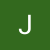# The Blind 75 Leetcode Series: Binary Tree Level Order TraversalPhoto by Chris Ried on Unsplash

Today, we are working on 102. Binary Tree Level Order Traversal.

Given the `root` of a binary tree, return the level order traversal of its nodes' values. (i.e., from left to right, level by level).

Rather simple problem. You can always ask about the empty tree if the 3rd example is not given. You can also confirm the output format. Other than that, I can’t really think of anything corner case related. Maybe to make sure they didn’t ask for a specific way to solve the problem or any additional constraint like limited space?

We can go right into it.

From Example 1, we can see that each level’s node values are grouped in a list. The result is a list of lists. This means we can simply track the nodes in some sort of table and then just return the values of the table.

`{    'level 1': [node1, node2, node3],    'level 2': [node4, node5],    'level 3': [node6....]}`

A dictionary does just that. We can just the levels as keys and nodes as values. When we get to a new level, create new key in the dictionary and append nodes to the values.

Now we just need to think of a way to traverse while carrying the level information. For each level, we have at most 2 children, and when we traverse down to that child level, we again have 2 children. This becomes a recursion.

We check for children for each node. If they exist, we recursively go down to the children while increasing the level notation.

So the recursive function would look like

`recursive(node, level, tracker):    # tracker[level].append(node)    # if node.left: call recursive on (node.left, level+1, tracker)    # if node.right: call recursive on (node.right, level+1, tracker)`

And have root level call the recursive with level=0.

To put everything to code:

`def recursive(root, tracker, level):    tracker[level].append(root.val)    if root.left:        recursive(root.left, tracker, level + 1)    if root.right:        recursive(root.right, tracker, level + 1)def levelOrder(root):    if not root:        return []    tracker = defaultdict(list)  # to avoid check for existence of a key    recursive(root, tracker, 0)    return tracker.values()`

With all nodes from the same level grouped together, we can simply call `tracker.values()` to get the final answer.

What’s the time complexity? Assuming we have N nodes, we will have to traverse through the entire tree, so we have O(N) for time complexity.

This is our DFS approach as we would keep going down the left child until we can’t. Then we come back and go to the right child.

Do we have a BFS approach?

Again we want to have a tracker, and we need a stack or queue to store the nodes we want to check.

We start by storing the root node in the stack, and then use a `while` loop to keep popping nodes from the stack until we can’t. We store the node in the tracker. Then we check for left child and right child. If we see one, store the child in the stack again with level = current_level + 1.

To put it into code:

`def levelOrder(root):    if not root:        return []    tracker = defaultdict(list)    stack = deque()    stack.append((root, 1))    while stack:        next_node, node_level = stack.popleft()        tracker[node_level].append(next_node.val)        if next_node.left:            stack.append((next_node.left, node_level + 1))        if next_node.right:            stack.append((next_node.right, node_level + 1))    return tracker.values()`

Because we are always popping from the beginning of the queue, the nodes are from the higher levels. This achieves the BFS.

The time complexity stays the same. We are still looking at O(N) for total complexity.

Tree traversal is a popular interview question for testing candidates for DFS and BFS. Make sure you know the difference between these two and how to put them into use. DFS drills down the tree until you can’t, and then you go to the neighbor. BFS checks for neighbors first and usually involves a queue/stack.

That’s it! Another problem down.